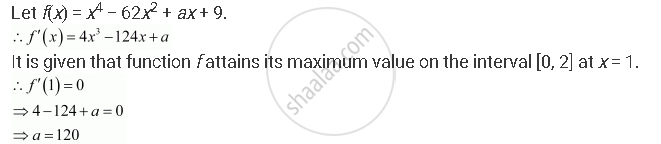Share

# It is Given that at X = 1, the Function X4− 62x2 + Ax + 9 Attains Its Maximum Value, on the Interval [0, 2]. Find the Value of A. - CBSE (Commerce) Class 12 - Mathematics

#### Question

It is given that at x = 1, the function x4− 62x2 + ax + 9 attains its maximum value, on the interval [0, 2]. Find the value of a.

#### SolutionHence, the value of a is 120.

Is there an error in this question or solution?

#### APPEARS IN

NCERT Solution for Mathematics Textbook for Class 12 (2018 to Current)
Chapter 6: Application of Derivatives
Q: 11 | Page no. 233

#### Video TutorialsVIEW ALL 

Solution It is Given that at X = 1, the Function X4− 62x2 + Ax + 9 Attains Its Maximum Value, on the Interval [0, 2]. Find the Value of A. Concept: Maxima and Minima.
S